Mobile QR Code1. (School of Electronic and Electrical Engineering, Kyungpook National University, Daegu 702-201, Korea)
2. (Korea Multi-purpose Accelerator Complex, Korea Atomic Energy Research Institute, Gyeongju 38180, Korea)
3. (Power Semiconductor Research Center, Korea Electrotechnology Research Institute, Changwon 51543, Korea)

Nanowire junctionless field-effect transistor, one-transistor dynamic random-access memory, work-function variation

## I. INTRODUCTION

Many studies are currently being conducted to determine if scaling can be employed to build more devices on the same chip size. However, short-channel effects (SCEs) occur in transistors as a side effect of scaling (1-4). Therefore, a method of increasing gate controllability using high-k (HK)/metal-gate (MG) is being used as a solution of SCE. Nevertheless, HK materials are incompatible with poly-Si gates for reasons such as fermi-level pinning and phonon scattering (5-7). Thus, metal materials, such as TiN, TaN, and MoN, are used instead of a poly-Si for the gate (8).

Owing to using MGs, fermi-level pinning and phonon scattering are alleviated. Moreover, MGs have different work-functions (WFs) depending on the grain orientation, causing a WF variation (WFV), which affects transfer characteristics, such as threshold voltage ( $V_{\text {th }}$ ), subthreshold swing (SS), on-current ( $I_{\text {on }}$ ), and off-current ( $I_{\text {off }}$ ). Recently, many scholars have investigated transfer characteristics, especially $V_{\text {th }}$ shift by WFV (9-11). WFV is a semiconductor-manufacturing issue that needs to be addressed. However, a study on the effect of WFV on gate-all-around junctionless field-effect transistor (GAA-JLFET)-based capacitorless dynamic random-access memory (DRAM) has not been reported yet.

In this study, not only the variation in transfer characteristics but also the variation in memory performances due to WFV were investigated for a GAA-JLFET-based capacitorless DRAM with a Si/SiGe heterostructure (12). Numerous samples were simulated; in addition, to the best of our knowledge, it is the first time an analysis of a capacitorless DRAM from the WFV perspective is performed.

## II. Device Structure and Simulation Method

Fig. 1 shows a three-dimensional (3-D) schematic view and cross-section view of the GAA-JLFET-based capacitorless DRAM. The Ge composition in SiGe of $0.2$ in the body region was determined from ref (12), which was an optimized value. The gate length $\left(L_{g}\right)$, underlap length ( $L_{\text {underlap }}$ ), nanowire radius $\left(R_{\text {nw }}\right)$, and gate dielectric $\left(\mathrm{HfO}_{2}\right)$ thicknesses $\left(T_{\mathrm{ox}}\right)$ were $70,15,10$, and $2 \mathrm{~nm}$, respectively, and the metal grain size $\left(G_{\text {size }}\right)$ was set to $10 \mathrm{~nm}$, a common measurement value (13,14). The role of the underlap structure was to reduce the electric field between the body and source/drain regions, which significantly affected the recombination/generation rate, which affected the improvement in retention time. For devices without WFV consideration, the body region was nearly depleted by the gate with a high WF of $5.0 \mathrm{eV}$ (12). However, the device considering WFV had a metal grain having WFs of $5.0$ and $4.4 \mathrm{eV}$ with $60 \%$ and $40 \%$ probability, respectively; it had a higher $I_{\text {off }}$ and a lower $V_{\text {th }}$ than the reference device. The doping concentrations of the source, body, and drain regions were $5 \times 10^{19}, 1 \times$ $10^{18}$, and $5 \times 10^{19} \mathrm{~cm}^{-3}$ ( $n$-type), respectively. The parameters for the proposed devices are listed in Table 1 .

The device design and analysis were performed using the Sentaurus 3-D Technology Computer-Aided Design (TCAD) simulation tool. The Sentaurus 3-D TCAD simulation tool was used to analyze the transfer characteristics and memory performances. Various physical models, such as the Shockley-Read-Hall recombination model, Fermi-Dirac statistical model, trap-assisted-tunneling model, Auger recombination model, nonlocal band-to-band tunneling model, doping-dependent and field-dependent mobility models, bandgap narrowing model, and quantum confinement effect were considered to obtain reliable results and maximize the simulation accuracy (15).

Fig. 1. 3-D schematic and cross-section view of the proposed GAA-JLFET based capacitorless DRAM cell using MoN metal gate material with randomly distributed WF values.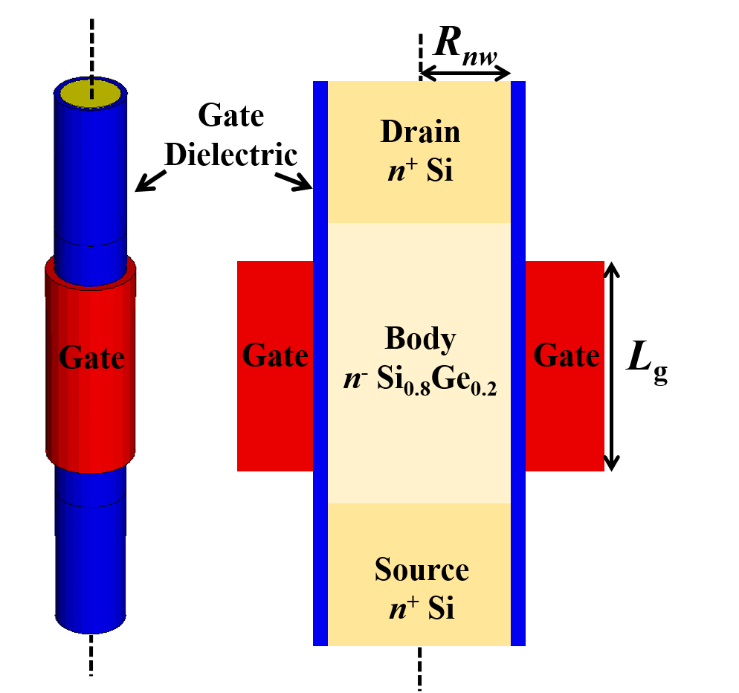Table 1. Device parameters of the proposed transistor used for simulation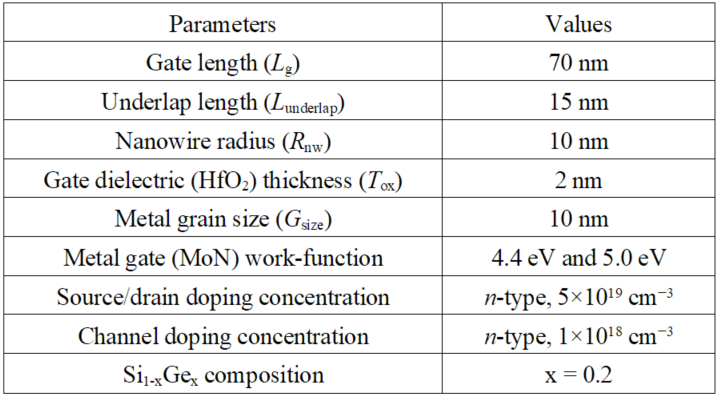## III. Results and Discussion

Fig. 2 shows the transfer curves of 200 samples of GAA-JLFET with the median data. To study the WFV effect, MoN MG, which has a high WF and can perform the memory operation of capacitorless DRAM, was used. The physical properties of various metals are summarized in Table 2 . The MoN comprises two types of grains, having different WFs depending on the grain orientation (e.g., WFs of $5.0$ and $4.4 \mathrm{eV}$ for $<110>$ and $<112>$, respectively). This phenomenon causes WFV in the GAA-JLFET, which fluctuates the transfer characteristics. Thus, the mean, standard deviation (SD), and relative SD (RSD) of the $V_{\text {th }}$ of the GAA-JLFET-

Fig. 2. Transfer characteristics for the 200 samples with ${G}_{\mathrm{size}}$ of 10 nm. The drain current is normalized by a nanowire diameter and ${V}_{\mathrm{th}}$ is defined by a constant-current method at 100 nA/μm (16).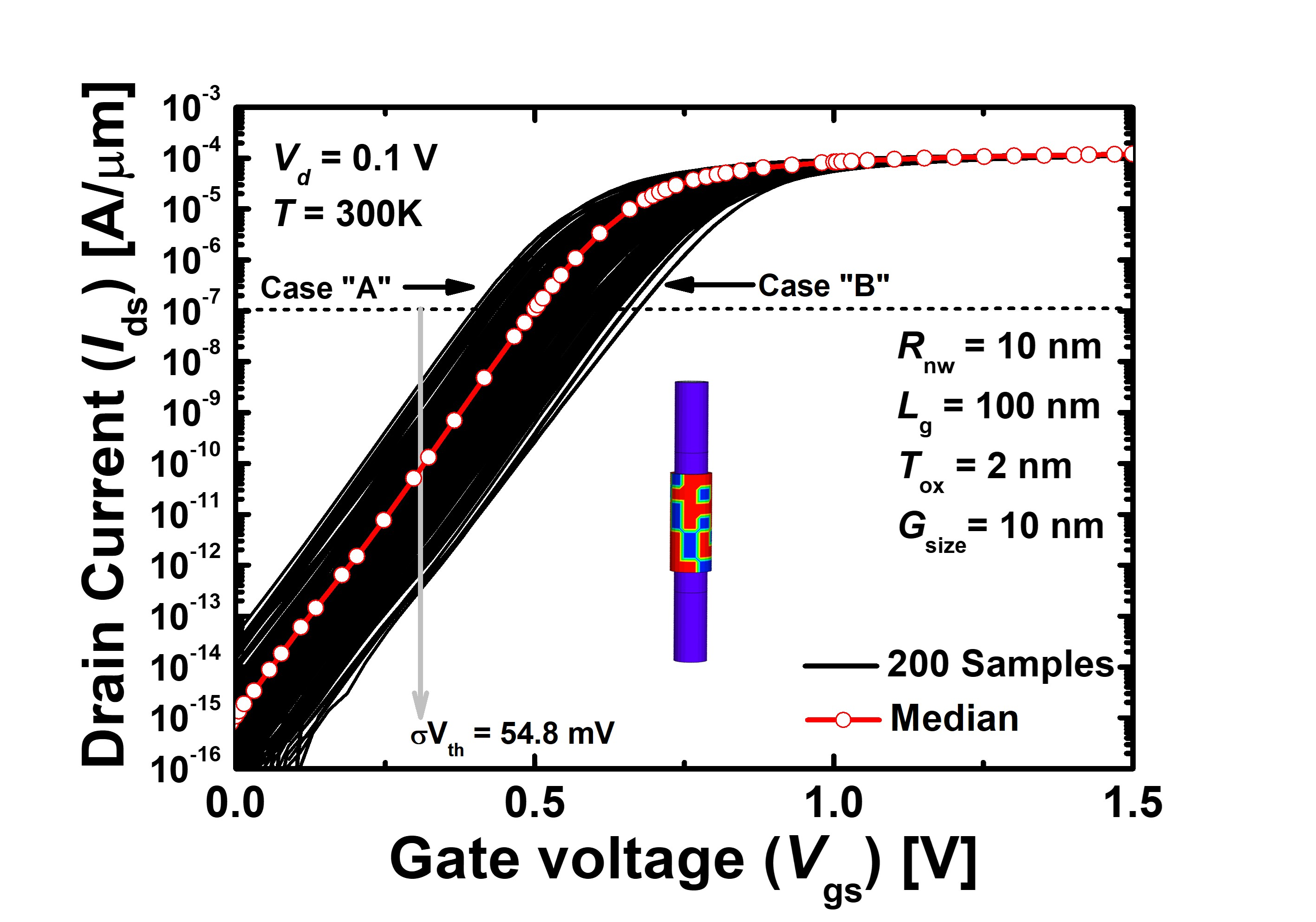Table 3. Comparison of mean, standard deviation (SD), and RSD of transfer characteristics of GAA-JLFET-based capacitorless DRAMs by WFV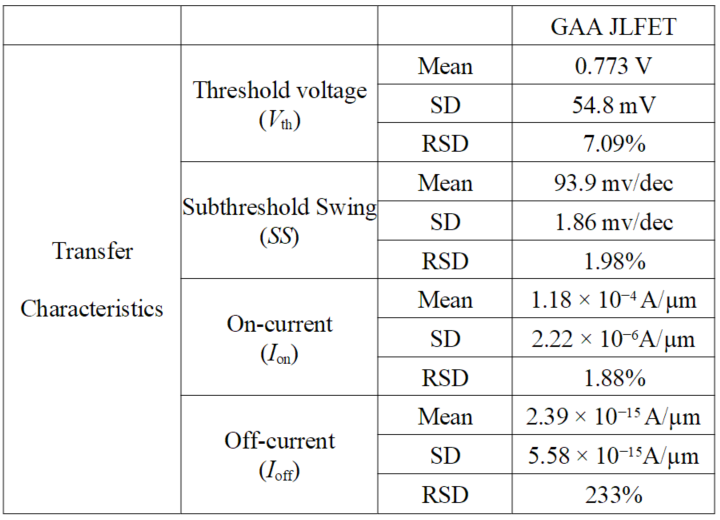based capacitorless DRAMs was $0.766 \mathrm{~V}, 54.3 \mathrm{mV}$, and $7.09 \%$, respectively. RSD is SD divided by the mean and multiplied by 100 . In other words, a large RSD means that the SD is relatively large compared with the mean and the dispersion is large. The RSD has no unit, so it is a coefficient of merit used to compare the spread of

Fig. 3. (a) Examples showing randomized WF values of grains in Cases “A” and “B” with MoN gate materials, (b) Energy band diagram of GAA-JLFET-based capacitorless DRAM. The energy band is extracted along X-X’ lines of GAA-JLFET across the center of the body region.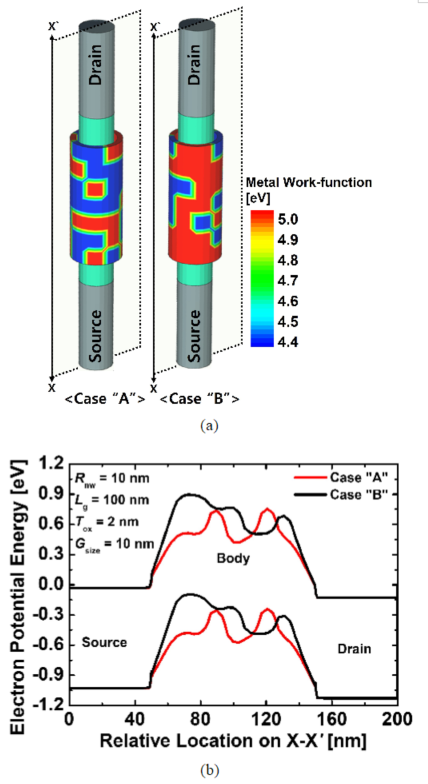datasets with different measurement units. The RSD of the $S S, I_{\mathrm{on}}$, and $I_{\mathrm{off}}$ of the GAA-JLFET-based capacitorless DRAMs were $1.98 \%$ and $1.88 \%$, and $233 \%$ respectively, indicating that the variation in $I_{\text {off }}$ by WFV was large, which can be a problem for designing highreliability devices. Detailed transfer characteristics of the GAA-JLFET-based capacitorless DRAMs by WFV are summarized in Table 3 .

Among the 200 randomly generated samples, the memory performances were analyzed using two extreme cases with the highest and lowest $V_{\text {th }}$ of GAA-JLFETs

Fig. 4. Hole density distribution of GAA-JLFET-based capacitorless DRAM at (a) state "1", (b) state "0", (c) Hole density distribution under the gate dielectric of the GAA-JLFET-based capacitorless DRAMs with different body materials at ${V}_{\mathrm{GS}}$ of 0 V and ${V}_{\mathrm{DS}}$ of 0 V. Hole density distribution is extracted along X-X’ lines of GAA-JLFET at the 2 nm below the gate oxide.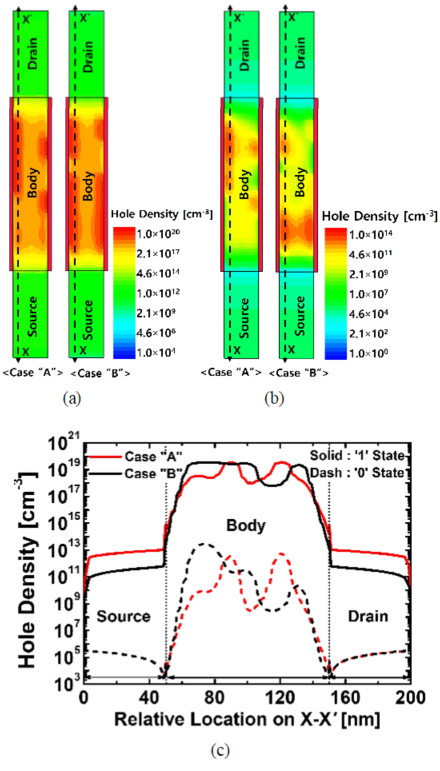(Cases "A" and "B," respectively) are shown in Fig. 3(a). Case "A" shows low WF grains were concentrated near the source/body and drain/body junctions. The low WF grains lowered the potential barrier between the source/body and drain/body junctions; thus, the transistor was turned on easily. Therefore, it had a low $V_{\text {th }}$. Contrariwise, Case "B" shows high WF grains were located at source/body and drain/body junctions. The high WF grains increased the potential barrier between source/body and drain/body junctions; thus, the transistor required more gate voltage to be turned on. Therefore, it had a high $V_{\text {th. }}$. As shown in Fig. 3(b), the energy barrier

Fig. 5. Read currents $I_{\mathrm{R} 1}$ and $I_{\mathrm{R} 0}$ as functions of $V_{\mathrm{G} \mathrm{R}}$ at the temperature of $358 \mathrm{~K}$ in Case "A" and Case "B".of Case "A" (i.e., red line) is lower than that of Case "B" (i.e., black line). Depending on the metal grain granularity, not only did transfer characteristics vary but also memory performances were affected by $V_{\text {th }}$. Fig. 4 (a) and (b) show the hole density distribution for states "1" and "0" of Cases "A" and "B." It shows that the distribution of hole density after write operation and erase operation, i.e., states "1" and " 0 ," when the electron potential energy is changed by WFV. For Case "A," compared with Case "B," the energy barrier is formed low at the source/channel and source/drain junctions due to the low wF distribution. Case "A" cannot form a physical barrier where holes can gather compared to Case "B." If the physical barrier is low, the hole density that can be confined becomes smaller, which in turn lowers the sensing margin among the memory performances. Case "B" has more holes than Case "A" in both states " 1 " and " 0 " [Fig. 4 (a)-(c)].

Fig. 5 shows the read currents at " 1 " and " 0 " States ( $I_{\mathrm{R} 1}$ and $I_{\mathrm{R} 0}$ ) as functions of gate voltage at the read operation ( $V_{\mathrm{G}_{-} \mathrm{R}}$ ). When $V_{\mathrm{G}_{-} \mathrm{R}}$ was $0.0$ and $0.2$ V, respectively, the GAA-JLFET-based capacitorless DRAM obtained an $I_{\mathrm{R} 1} / I_{\mathrm{R} 0}$ ratio of about $10^{2}$ and $10^{4}$, respectively, a much smaller value than the existing $I_{\mathrm{R} 1} / I_{\mathrm{R} 0}$ ratio of $10^{8}$(12). The reason for this phenomenon is that the energy barrier is lowered by wFV, and the capability to confine the hole is weakened; thus, the hole density in the body region is lowered accordingly. As $V_{\mathrm{G}_{-} \mathrm{R}}$ increased, the $I_{\mathrm{R} 1} / I_{\mathrm{R} 0}$ ratio and sensing margin decreased because as $V_{\mathrm{G}_{-} \mathrm{R}} \mathrm{~ i n c r e a s e d , ~ a ~ c h a n n e l ~ w a s}$ formed, even at state “0.” The sensing margin is the

Fig. 6. (a) Read currents $I_{\mathrm{R} 1}$ and $I_{\mathrm{R} 0}$ as functions of hold time at the temperatures of $358 \mathrm{~K}$, (b) Comparison of sensing margins as functions of hold time between Cases "A" and "B" at $358 \mathrm{~K}$ temperature.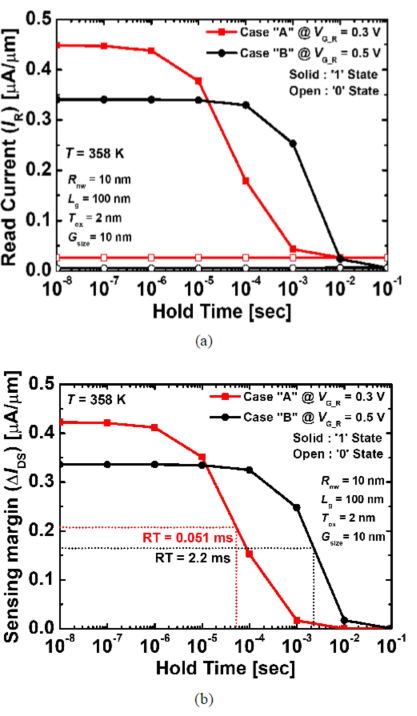difference between $I_{R 1}$ and $I_{R 0}$. As shown in Fig. 5, the sensing margins of Cases "A" and "B" were $0.42$ and $0.34 \mu \mathrm{A} / \mu \mathrm{m}$ at $V_{\mathrm{G}_{-} \mathrm{R}}$ of $0.3$ and $0.5 \mathrm{~V}$, respectively. When considering the gate voltage where $I_{\mathrm{R} 1} / I_{\mathrm{R} 0}$ is the maximum, $0.0$ and $0.2 \mathrm{~V}$ should be applied. $V_{\mathrm{G}_{-} \mathrm{R}}$ at $0.0$ and $0.2 \mathrm{~V}$ have a high $I_{\mathrm{R} 1} / I_{\mathrm{R} 0}$ ratio but a very poor sensing margin. Therefore, $0.3$ and $0.5 \mathrm{~V}$ were selected as $V_{\mathrm{G}_{-} \mathrm{R}}$ because they did not only a high sensing margin but also easily distinguishable due to high $I_{R 1} I_{R 0}$ ratio. There were more hole densities in Case "B," but the sensing margin of Case "A" was $0.42 \mu \mathrm{A} / \mu \mathrm{m}$, larger than that of Case "B" $(0.34 \mu \mathrm{A} / \mu \mathrm{m})$, which was because $V_{\mathrm{G}_{-} \mathrm{R}}$ and hole density significantly affected the sensing margin. In summary, the storage number of holes was larger in

Fig. 7. (a) Read currents $I_{\mathrm{R} 1}$ and $I_{\mathrm{R} 0}$ as functions of hold time at the temperatures of $358 \mathrm{~K}$, (b) Comparison of sensing margins as functions of hold time between Cases "A" and "B" at $358 \mathrm{~K}$ temperature. The Shockley-Read-Hall recombination rate is extracted along X-X' lines of GAA-JLFET at the $2 \mathrm{~nm}$ below the gate oxide.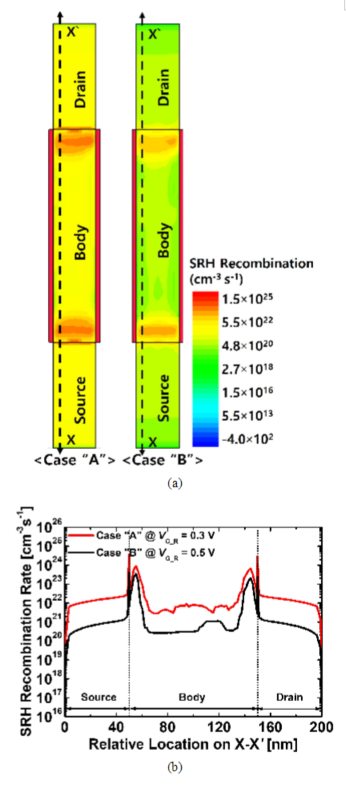Case "B,"' but the sensing margin was higher in Case "A" due to the low gate voltage.

Fig. 6(a) and (b) show the $I_{\mathrm{R}}$ at " 1 " and " 0 " states and the sensing margin as functions of hold time when the $V_{\mathrm{G}_{-} \mathrm{R}}$ of Cases "A" and "B" was $0.3$ and $0.5 \mathrm{~V}$, respectively. The retention time is the time for the sensing margin to drop below $50 \%$ of its value (17). In a previous paper (12), a retention time of more than $10 \mathrm{~ms}$ was obtained when WFV was not considered. However, short retention times of $0.051 \mathrm{~ms}$ and $2.2 \mathrm{~ms}$ were obtained for Cases "A" and "B," respectively.

WFV is also detrimental to memory performances because the low WF of the metal grains directly affects the recombination/generation rate, which is a significant parameter of memory performances when located at the source/body and drain/body junctions [Fig. 7(a)]. In Case "A," owing to the lower WF than in Case "B," about 10 times more recombination occurred in the junction region based on the peak [Fig. 7(b)]. This caused the hole held in the body region to reduce more rapidly according to the hold time, reducing the retention time, which is the hole holding capacity. Consequently, compared with the performances of the GAA-JLFET-based capacitorless DRAM without WFV, GAA-JLFET-based capacitorless DRAM with WFV significantly degraded retention time because of metal grain variation. Without WFV, the current ratio was $10^{8}$, and the retention time was more than $10 \mathrm{~ms}$; meanwhile, with WFV, the current ratio was $10^{2}$ and $10^{4}$, and the retention time decreases to $0.051 \mathrm{~ms}$ and $2.2 \mathrm{~ms}$, respectively. This result shows that WFV impacts not only the transfer characteristics in GAAJLFET but also memory performances; in addition, it can adversely affect the reliability of the memory device.

## IV. CONCLUSIONS

This paper presents transfer characteristics and memory performances variation caused by WFV in the metal gate of capacitorless DRAM cell based on a GAAJLFET. Due to the randomly distribution of metal gate grain orientation, 200 samples have different transfer characteristics such as $V_{\text {th }}, S S, I_{\text {off }}$, and $I_{\text {on. }}$ The RSD of $V_{\text {th }}, S S, I_{\text {off, }}$ and $I_{\text {on }} 7.09 \%, 1.98 \%, 1.88 \%$, and $233 \%$, respectively. In terms of the memory performances, the GAA-JLFET based capacitorless DRAM with considered WFV obtained $I_{\mathrm{R} 1} / I_{\mathrm{R} 0}$ ratio of about $10^{2}$ and $10^{4}$, respectively. This is a much smaller value than the previous experiment $I_{\mathrm{R} 1} / I_{\mathrm{R} 0}$ ratio of $10^{8}$ which does not take WFV into account. Additionally, long retention time more than 10 ms were obtained for retention time when WFV was not considered. However, take WFV into account, poor retention times of 0.051 ms and 2.2 ms were obtained, respectively, which differ by more than 5 to 100 times depending on the samples. Consequently, WFV has impact on the transfer characteristics in GAA-JLFET based capacitorless DRAM. It also significantly affects memory performances such as sensing margin and retention time.

### ACKNOWLEDGMENTS

This work was supported by the National Research Foundation of Korea (NRF) grant funded by the Korea government (MSIT) (No. NRF-2020R1A2C1005087). This study was supported by the BK21 FOUR project funded by the Ministry of Education, Korea (4199990113966), by the Ministry of Trade, Industry & Energy (MOTIE) (10080513) and Korea Semiconductor Research Consortium (KSRC) support program for developing the future semiconductor devices. This research was supported by Basic Science Research Program through the National Research Foundation of Korea (NRF) funded by the Ministry of Education (NRF-2021R1A6A3A13039927). This research was supported by National R&D Program through the National Research Foundation of Korea (NRF) funded by Ministry of Science and ICT (2021M3F3A2A03017764). The EDA tool was supported by the IC Design Education Center (IDEC), Korea.

### REFERENCES

1
Veeraraghavan Surya, Fossum. Jerry G., Mar 1989, Short-channel effects in SOI MOSFETs., IEEE Transactions on Electron Devices, Vol. 36, No. 3, pp. 522-5282
Zhang J., Yuan J. S., Ma Y., Nov 2000, Modeling short channel effect on high-k and stacked-gate MOSFETs., Solid-state electronics, Vol. 44, No. 11, pp. 2089-20913
Tsormpatzoglou A., Dimitriadis C. A., Clerc R., Rafhay Q., Pananakakis G., Ghibaudo G., Aug 2007, Semi-analytical modeling of short-channel effects in Si and Ge symmetrical double-gate MOSFETs., IEEE Transactions on Electron devices, Vol. 54, No. 8, pp. 1943-19524
Khan M. U., Arshad A., Ume S., Bukhari R., Samer A., Tariq F., Aug 2021, Nanoscale CMOS Scaling Trends, Challenges and Their Solutions., Vol. icame21 international conference on advances in mechanical engineering, No. pakistan, pp. 1-105
Takeuchi H., Wong H. Y., Ha D., King T. J., Dec 2004, Impact of oxygen vacancies on high-κ gate stack engineering., IEEE International Electron Devices Meeting, pp. 829-8326
Kar S., Jul 2013, High permittivity gate dielectric materials, Heidelberg: Springer, Vol. 437
Shin C., Jun 2016, Variation-aware advanced CMOS devices and SRAM, Dordrecht: Springer, Vol. 568
Dadgour H., Endo K., De V., Banerjee K., Dec 2008, Modeling and analysis of grain-orientation effects in emerging metal-gate devices and implications for SRAM reliability, IEEE International Electron Devices Meeting, pp. 1-49
Nam H., Shin C., Jun 2014, Impact of current flow shape in tapered (versus rectangular) FinFET on t hreshold voltage variation induced by work-function variation, IEEE Transactions on Electron Devices, Vol. 61, No. 6, pp. 2007-201110
Lee Y., Nam H., Park J. D., Shin C., Jul 2015, Study of Work-Function Variation for High-κ/Metal-Gate Ge-Source Tunnel Field-Effect Transistors.”, IEEE Transactions on Electron Devices, Vol. 62, No. 7, pp. 2143-214711
Guan Y., Carrillo-Nuñez H., Georgiev V. P., Asenov A., Liang F., Li Z., Chen H., Apr 2021, Quantum simulation investigation of work-function variation in nanowire tunnel FETs.”, Nanotechnology, Vol. 32, No. 15, pp. 150001-1-150001-812
Yoon Y. J., Lee J. S., Kim D. S., Lee S. H., Kang I. M., Dec 2020, One-transistor dynamic random-access memory based on gate-all-around junction-less field-effect transistor with a Si/SiGe heterostructure.”, Electronics, Vol. 9, No. 12, pp. 2134-1-2134-1213
Bouaouina B., Besnard A., Abaidia S. E., Airoudj A., Bensouici F., Jan 2018, Correlation between mechanical and microstructural properties of molybdenum nitride thin films deposited on silicon by reactive RF magnetron discharge.”, Surface and Coatings Technology, Vol. 333, pp. 32-3814
Anitha V. P., Major S., Chandrashekharam D., Bhatnagar M., Feb 1996, Deposition of molybdenum nitride thin films by rf reactive magnetron sputtering.”, Surface and Coatings Technology, Vol. 79, vol. 1-3, pp. 50-5415
Sentaurus User's Manual , 2016, Version L-2016.03, Synopsys, Mountain View, CA, USA16
Kim H. W., Kwon D., Sep 2020, Impact of body-biasing for negative capacitance field-effect transistor., Journal of Physics Communications, Vol. 4, No. 9, pp. 095019-17
Cho M. H., Shin C., Liu T. J. K., Sep. 2009, Convex channel design for improved capacitorless DRAM retention time., International Conference on Simulation of Semiconductor Processes and Devices IEEE, pp. 1-4## Author

Sang Ho Lee received the B.Sc. degree in electronics engineering from the School of Electronics Engineering (SEE), Kyungpook National University (KNU), Daegu, South Korea, in 2019, where he is currently pursuing the Ph.D. in School of Electronic and Electrical Engineering, Kyungpook National University (KNU), Daegu, South Korea.

His research interests include the design, fabrication, and characterization of gate-all-around logic devices and capacitor-less 1T-DRAM transistors.

Young Jun Yoon received the B.S. and Ph.D. degrees in electronics engineering from Kyungpook National University, Daegu, Korea, in 2013 and 2019, respectively.

He is currently postdoctoral researcher with Korea Multi-purpose Accelera-tor Complex, Korea Atomic Energy Research Institute (KAERI).

His research interests include design, fabrication, and characterization of logic transistor and memory.

Jae Hwa Seo received the B.S. and Ph.D. degrees in Electronics Engi-neering from the School of Electronics Engineering, Kyungpook National University (KNU), Daegu, Korea, in 2012, 2018.

He worked as a Post Doc. in electrical engineering from School of Electrical Engineering and Computer Science (EECS), Seoul National University (SNU), Seoul, Korea, in 2018 to 2019.

Now, he has worked as reseacher at Power Semiconductor Research Center, Korea Electrotechnology Research Institute.

His research interests include the design, fabrication and characterization of V-NAND/1T-DRAM devices, nano-scale CMOS, tunneling FETs, and compound/silicon based transistors.

Min Su Cho received a B.Sc. degree in computer engineering from the College of Electrical and Computer Engineering, Chungbuk National University (CBNU), Cheongju, South Korea, in 2015, and an M.Sc. degree from the School of Electronics Engineering (SEE), Kyungpook National University (KNU), and Ph.D. degree in Electronics Engineering from the School of Electronic and Electrical Engineering.

He is currently postdoctoral researcher with KNU.

His research interests include the design, fabrication, and characterization of compound CMOS, tunneling FETs, and III–V compound transistors.

Jin Park received a B.Sc. degree in electronic engineering from the School of Electronics Engineering (SEE), Kyungpook National University (KNU), Daegu, South Korea, in 2020, where she is currently pursuing the M.Sc. degree in School of Electronic and Electrical Engineering, Kyungpook National University (KNU), Daegu, South Korea.

Her research interests include the design, fabrication, and characterization of gate-all-around logic devices and capacitor-less 1T-DRAM transistors.

Hee Dae An received the B.Sc. degree in School of Electronic Engineering, Kumoh National Institute of Techology (KIT), Gumi, South Korea, in 2019, where he is currently pursuing the M.Sc. degree in School of Electronic and Electrical Engineering, Kyungpook National University (KNU), Daegu, South Korea.

His research interests include the design, fabrication, and characterization of capacitor-less 1T-DRAM transistors and vertical GaN power devices.

So Ra Min received the B.Sc. degree in Electronic Engineering from the School of Electronics Engineering, Yeungnam University (YU), Gyeong-san, North Gyeongsang, South Korea, in 2020, where she is currently pursuing the M.Sc. degree in school of Electronic and Electrical Engineering.

Her research interests include the design, fabrication, and characterization of GaN devices and capacitor-less 1T-DRAM transistors.

Geon Uk Kim received a B.Sc. degree in electronic engineering from the School of Electronics Engi-neering (SEE), Kyungpook National University (KNU), Daegu, South Korea, in 2021, where he is currently pursuing the M.Sc. degree in School of Electronic and Electrical Engineering, Kyungpook National University (KNU), Daegu, South Korea.

His research interests include the design, fabrication, and characterization of GaN devices and capacitor-less 1T-DRAM transistors.

In Man Kang received the B.S. degree in electronic and electrical engineering from School of Electronics and Electrical Engi-neering, Kyungpook National University (KNU), Daegu, Korea, in 2001, and the Ph.D. degree in electrical engineering from School of Electrical Engineering and Computer Science (EECS), Seoul National University (SNU), Seoul, Korea, in 2007.

He worked as a teaching assistant for semiconductor process education from 2001 to 2006 at Inter-university Semiconductor Research Center (ISRC) in SNU.

From 2007 to 2010, he worked as a senior engineer at Design Technology Team of Samsung Electronics Company.

In 2010, he joined KNU as a full-time lecturer of the School of Electronics Engineering (SEE).

Now, he is currently working as a professor.

His current research interests include CMOS RF modeling, silicon nanowire devices, tunneling transistor, low-power nano CMOS, and III-V compound semiconductors.

He is a member of IEEE EDS.